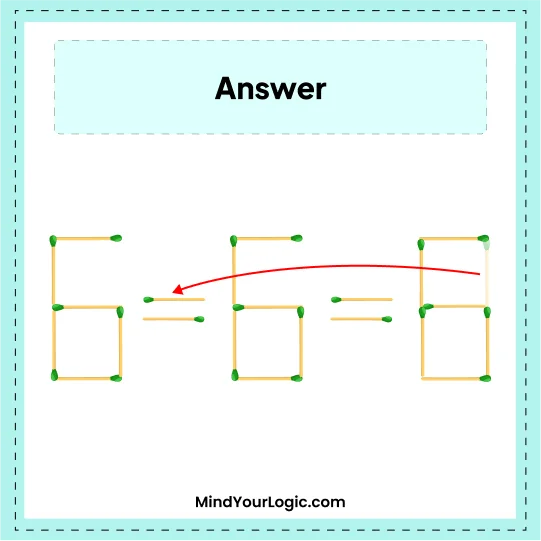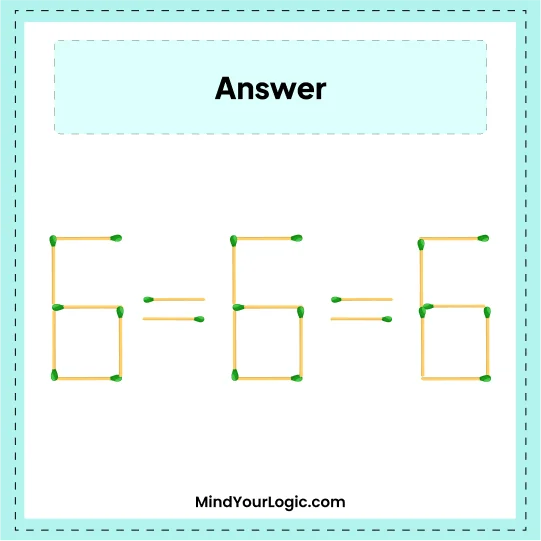# 6-6=8 - Matchstick Equation

###### 38.Matchstick Puzzles
`	Move One Matchstick to correct the following equation.`•

Explanation :

``` Just move one matchstick from number 8 and place it below - the sign which becomes =.
So, we will get an equation as 6 = 6 = 6.```